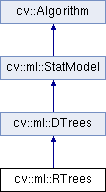OpenCV  3.2.0 Open Source Computer Vision
cv::ml::RTrees Class Referenceabstract

The class implements the random forest predictor. More...

#include "ml.hpp"

Inheritance diagram for cv::ml::RTrees:## Public Member Functions

virtual int getActiveVarCount () const =0

virtual bool getCalculateVarImportance () const =0

virtual TermCriteria getTermCriteria () const =0

virtual Mat getVarImportance () const =0

virtual void setActiveVarCount (int val)=0

virtual void setCalculateVarImportance (bool val)=0

virtual void setTermCriteria (const TermCriteria &val)=0Public Member Functions inherited from cv::ml::DTrees
virtual int getCVFolds () const =0

virtual int getMaxCategories () const =0

virtual int getMaxDepth () const =0

virtual int getMinSampleCount () const =0

virtual const std::vector< Node > & getNodes () const =0
Returns all the nodes. More...

virtual cv::Mat getPriors () const =0
The array of a priori class probabilities, sorted by the class label value. More...

virtual float getRegressionAccuracy () const =0

virtual const std::vector< int > & getRoots () const =0
Returns indices of root nodes. More...

virtual const std::vector< Split > & getSplits () const =0
Returns all the splits. More...

virtual const std::vector< int > & getSubsets () const =0
Returns all the bitsets for categorical splits. More...

virtual bool getTruncatePrunedTree () const =0

virtual bool getUse1SERule () const =0

virtual bool getUseSurrogates () const =0

virtual void setCVFolds (int val)=0

virtual void setMaxCategories (int val)=0

virtual void setMaxDepth (int val)=0

virtual void setMinSampleCount (int val)=0

virtual void setPriors (const cv::Mat &val)=0
The array of a priori class probabilities, sorted by the class label value. More...

virtual void setRegressionAccuracy (float val)=0

virtual void setTruncatePrunedTree (bool val)=0

virtual void setUse1SERule (bool val)=0

virtual void setUseSurrogates (bool val)=0Public Member Functions inherited from cv::ml::StatModel
virtual float calcError (const Ptr< TrainData > &data, bool test, OutputArray resp) const
Computes error on the training or test dataset. More...

virtual bool empty () const
Returns true if the Algorithm is empty (e.g. in the very beginning or after unsuccessful read. More...

virtual int getVarCount () const =0
Returns the number of variables in training samples. More...

virtual bool isClassifier () const =0
Returns true if the model is classifier. More...

virtual bool isTrained () const =0
Returns true if the model is trained. More...

virtual float predict (InputArray samples, OutputArray results=noArray(), int flags=0) const =0
Predicts response(s) for the provided sample(s) More...

virtual bool train (const Ptr< TrainData > &trainData, int flags=0)
Trains the statistical model. More...

virtual bool train (InputArray samples, int layout, InputArray responses)
Trains the statistical model. More...Public Member Functions inherited from cv::Algorithm
Algorithm ()

virtual ~Algorithm ()

virtual void clear ()
Clears the algorithm state. More...

virtual String getDefaultName () const

virtual void read (const FileNode &fn)
Reads algorithm parameters from a file storage. More...

virtual void save (const String &filename) const

virtual void write (FileStorage &fs) const
Stores algorithm parameters in a file storage. More...

## Static Public Member Functions

static Ptr< RTreescreate ()Static Public Member Functions inherited from cv::ml::DTrees
static Ptr< DTreescreate ()
Creates the empty model. More...Static Public Member Functions inherited from cv::ml::StatModel
template<typename _Tp >
static Ptr< _Tp > train (const Ptr< TrainData > &data, int flags=0)
Create and train model with default parameters. More...Static Public Member Functions inherited from cv::Algorithm
template<typename _Tp >
static Ptr< _Tp > load (const String &filename, const String &objname=String())
Loads algorithm from the file. More...

template<typename _Tp >
static Ptr< _Tp > loadFromString (const String &strModel, const String &objname=String())
Loads algorithm from a String. More...

template<typename _Tp >
static Ptr< _Tp > read (const FileNode &fn)
Reads algorithm from the file node. More...Public Types inherited from cv::ml::DTrees
enum  Flags {
PREDICT_AUTO =0,
PREDICT_SUM =(1<<8),
PREDICT_MAX_VOTE =(2<<8),
}Public Types inherited from cv::ml::StatModel
enum  Flags {
UPDATE_MODEL = 1,
RAW_OUTPUT =1,
COMPRESSED_INPUT =2,
PREPROCESSED_INPUT =4
}Protected Member Functions inherited from cv::Algorithm
void writeFormat (FileStorage &fs) const

## Detailed Description

The class implements the random forest predictor.

Random Trees

## § create()

 static Ptr cv::ml::RTrees::create ( )
static

Creates the empty model. Use StatModel::train to train the model, StatModel::train to create and train the model, Algorithm::load to load the pre-trained model.

## § getActiveVarCount()

 virtual int cv::ml::RTrees::getActiveVarCount ( ) const
pure virtual

The size of the randomly selected subset of features at each tree node and that are used to find the best split(s). If you set it to 0 then the size will be set to the square root of the total number of features. Default value is 0.

setActiveVarCount

## § getCalculateVarImportance()

 virtual bool cv::ml::RTrees::getCalculateVarImportance ( ) const
pure virtual

If true then variable importance will be calculated and then it can be retrieved by RTrees::getVarImportance. Default value is false.

setCalculateVarImportance

## § getTermCriteria()

 virtual TermCriteria cv::ml::RTrees::getTermCriteria ( ) const
pure virtual

The termination criteria that specifies when the training algorithm stops. Either when the specified number of trees is trained and added to the ensemble or when sufficient accuracy (measured as OOB error) is achieved. Typically the more trees you have the better the accuracy. However, the improvement in accuracy generally diminishes and asymptotes pass a certain number of trees. Also to keep in mind, the number of tree increases the prediction time linearly. Default value is TermCriteria(TermCriteria::MAX_ITERS + TermCriteria::EPS, 50, 0.1)

setTermCriteria

## § getVarImportance()

 virtual Mat cv::ml::RTrees::getVarImportance ( ) const
pure virtual

Returns the variable importance array. The method returns the variable importance vector, computed at the training stage when CalculateVarImportance is set to true. If this flag was set to false, the empty matrix is returned.

## § setActiveVarCount()

 virtual void cv::ml::RTrees::setActiveVarCount ( int val )
pure virtual

## § setCalculateVarImportance()

 virtual void cv::ml::RTrees::setCalculateVarImportance ( bool val )
pure virtual

## § setTermCriteria()

 virtual void cv::ml::RTrees::setTermCriteria ( const TermCriteria & val )
pure virtual